##### Actions

The problem of finding a function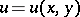which satisfies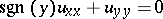(a1)

in a mixed domain that is simply connected and bounded by a Jordan (non-self-intersecting) "elliptic" arc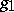(for) with end-points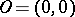andand by the "real" characteristics (for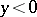)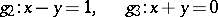of the Bitsadze–Lavrent'ev equation (a1), which satisfy the characteristic equationand meet at the point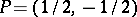, and which assumes prescribed continuous boundary values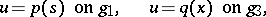(a2)

whereis the arc length reckoned from the pointand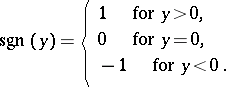Consider the aforementioned domain (denoted by). Then a functionis a regular solution of the Bitsadze–Lavrent'ev problem if it satisfies the following conditions:

1)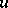is continuous in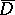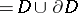,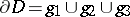;

2)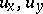are continuous in(except, possibly, at the points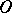and, where they may have poles of order less than, i.e., they may tend to infinity with order less thanas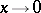and);

3),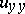are continuous in(except possibly on, where they need not exist);

4)satisfies (a1) at all points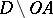(i.e.,without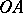);

5)satisfies the boundary conditions (a2).

Consider the normal curve (of Bitsadze–Lavrent'ev)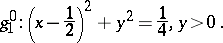Note that it is the upper semi-circle and can also be given by (the upper part of)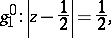where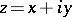. The curvecontainsin its interior.

The idea of A.V. Bitsadze and M.A. Lavrent'ev for finding regular solutions of the above problem is as follows. First, solve the problem N (in,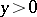). That is, find a regular solution of equation (a1) satisfying the boundary conditions: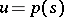on;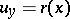on, where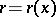is continuous for,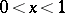, and may tend to infinity of order less thanasand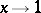.

Secondly, solve the Cauchy–Goursat problem (in,). That is, find a regular solution of (a1) satisfying the boundary conditions: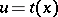on;on, where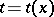is continuous for,, and may tend to infinity of order less thatasand.

Finally, take into account the boundary condition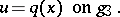Therefore, one has a Goursat problem (in,) for (a1) with boundary conditions:on;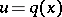on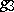.

Several extensions and generalizations of the above boundary value problem of mixed type have been established [a3], [a4], [a5], [a6], [a7], [a8]. These problems are important in fluid mechanics (aerodynamics and hydrodynamics, [a1], [a2]).